Courses

# Test: Past 28 Years Questions: Atoms

## 40 Questions MCQ Test Physics For JEE | Test: Past 28 Years Questions: Atoms

Description
This mock test of Test: Past 28 Years Questions: Atoms for NEET helps you for every NEET entrance exam. This contains 40 Multiple Choice Questions for NEET Test: Past 28 Years Questions: Atoms (mcq) to study with solutions a complete question bank. The solved questions answers in this Test: Past 28 Years Questions: Atoms quiz give you a good mix of easy questions and tough questions. NEET students definitely take this Test: Past 28 Years Questions: Atoms exercise for a better result in the exam. You can find other Test: Past 28 Years Questions: Atoms extra questions, long questions & short questions for NEET on EduRev as well by searching above.
QUESTION: 1

### What is the radius of iodine atom (At. no. 53,mass no. 126)          

Solution:

53 electrons in iodine atom are distributed as
2, 8, 18, 18, 7
∴ n = 5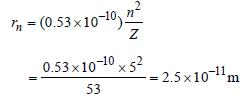QUESTION: 2

### The ionisation energy of hydrogen atom is 13.6eV, the ionisation energy of helium atom wouldbe     

Solution:

E ∝ Z2 and Z for helium = 2
∴ (E)He  = 4x13.6 = 54.4 eV

QUESTION: 3

### To explain his theory, Bohr used 

Solution:

Bohr used conservation of angular
momentum.

QUESTION: 4

The ground state energy of H-atom 13.6 eV. Theenergy needed to ionize H-atom from its secondexcited state. 

Solution:

Second excited state corresponds to n = 3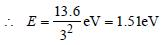QUESTION: 5

In terms of Bohr radius a0, the radius of the second Bohr orbit of a hydrogen atom is given by       

Solution:

As r ∝ n2 , therefore, radius of 2nd
Bohr’s orbit = 4 a0.

QUESTION: 6

The ionization energy of hydrogen atom is 13.6eV. Following Bohr’s theory, the energycorresponding to a transition between 3rd and4th orbit is 

Solution:

According to Bohr's theory,
En​= −13.6​/ n2
∴E3​=−13.6​/32=−13.6/9​=−1.51eV
E4​=−13.6/42​=−13.6/16​=−0.85eV
Energy corresponding to a transition between the 3rd and 4th orbit =E4​−E3​=−0.85−(−1.51) =0.66eV
Hence, the energy corresponding to a transition between the 3rd and 4th orbit is 0.66eV.

QUESTION: 7

Which source is associated with a line emissionspectrum ? 

Solution:

Neon street sign is a source of line emission
spectrum.

QUESTION: 8

In Rutherford scattering experiment, what willbe the correct angle for α-scattering for an impactparameter, b = 0 ? 

Solution:

Impact parameter for Rutherford scattering
experiment,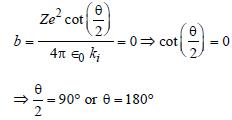QUESTION: 9

The radius of hydrogen atom in its ground stateis 5.3 × 10–11 m. After collision with an electron itis found to have a radius of 21.2 × 10–11 m. Whatis the principal quantum number n of the finalstate of the atom           

Solution:

r ∝ n2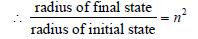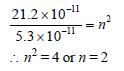QUESTION: 10

The spectrum obtained from a sodium vapourlamp is an example of 

Solution:

A spectrum is observed, when light coming
directly from a source is examined with a
spectroscope. Therefore spectrum obtained
from a sodium vapour lamp is emission
spectrum.

QUESTION: 11

When a hydrogen atom is raised from the groundstate to an excited state, 

Solution: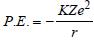and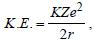where,
r is the radius of orbit which increases as we
move from ground to an excited state.
Therefore, when a hydrogen atom is raised
from the ground state, it increases the value
of r. As a result of this, P.E. increases
(decreases in negative) and K.E. decreases.

QUESTION: 12

If the threshold wavelength for a certain metal is 2000 Å, then the work-function of the metal is           

Solution:

Threshold wavelength (λ) = 2000 Å = 2000 ×
10–10 m. Work function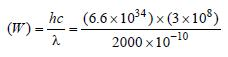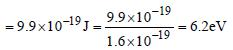QUESTION: 13

When hydrogen atom is in its first excited level,its radius is      

Solution: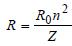Radius in ground state =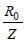Radius in first excited state =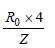(∵ n =2)
Hence, radius of first excited state is four
times the radius in ground state.

QUESTION: 14

In the Bohr model of a hydrogen atom, the centripetal force is furnished by the coulomb attraction between the proton and the electron. If a0 is the radius of the ground state orbit, m is the mass, e is the charge on the electron and ε0 is the vacuum  ermittivity, the speed of the electron is                 

Solution:

Centripetal force = Coulombian force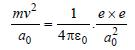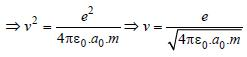QUESTION: 15

Who indirectly determined the mass of theelectron by measuring the charge of the electron?     

Solution:

Explanation : The mass of the electron is discovered by  the Millikan by the oil drop experiment.

E = mg

=> qE = mg

q/m = g/E

Therefore, charge was quantised.

QUESTION: 16

When an electron jumps from the fourth orbit tothe second orbit, one gets the      

Solution:

When the electron drops from any orbit to
second orbit, then wavelength of line
obtained belongs to Balmer series.

QUESTION: 17

Which of the following transitions in a hydrogenatom emits the photon of highest frequency?         

Solution: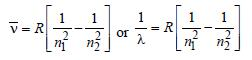Frequency,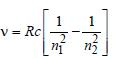Note : See the greatest energy difference and
also see that the transition is from higher to
lower energy level. Hence, it is highest in
case of n = 2 to n = 1.

QUESTION: 18

An electron changes its position from orbit n = 2 to the orbit n = 4 of an atom. The wavelength of the emitted radiations is (R = Rydberg’s constant)

Solution: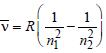, where n1 = 2, n2 = 4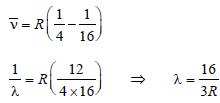QUESTION: 19

The energy of hydrogen atom in nth orbit is En, then the energy in nth orbit of single ionised helium atom will be 

Solution:

We have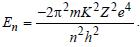For helium
Z = 2. Hence requisite answer is 4En.

QUESTION: 20

J.J. Thomson’s cathode-ray tube experimentdemonstrated that  

Solution:

Cathode rays are streams of negatively
charged ions

QUESTION: 21

In which of the following systems will the radiusof the first orbit (n = 1) be minimum ? 

Solution: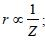Z(=3) is maximum for Li2+.

QUESTION: 22

The Bohr model of atoms   

Solution:

In Bohr’s model, angular momentum is
quantised i.e  =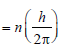QUESTION: 23

The Bohr model of atomsEnergy E of a hydrogen atom with principalquantum number n is given by E = – 13.6/n2 eV.The energy of photon ejected when the electronjumps from n = 3 state to n = 2 state of hydrogenis approximately 

Solution:

ΔE=E3​−E2​
=(−13.6/32)​+ (13.6​/22)
=13.6[(1/4)​−(1/9)​]
eV=1.9eV

QUESTION: 24

The half life of radium is about 1600 years. Of 100 g of radium existing now, 25 g will remainunchanged after 

Solution: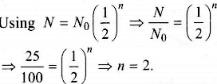The total time in which radium change to 25 g is

= 2 x 1600

= 3200 yr.

QUESTION: 25

The total energy of an electron in the firstexcited state of hydrogen atom is about –3.4eV. Its kinetic energy in this state is 

Solution: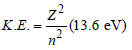Mechanical energy =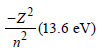∴ K.E. in 2nd orbital for hydrogen
= – Mechanical energy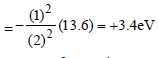QUESTION: 26

Ionization potential of hydrogen atom is 13.6eV.Hydrogen atoms in the ground state are excitedby monochromatic radiation of photon energy12.1 eV. According to Bohr’s theory, the spectrallines emitted by hydrogen will be 

Solution:

Energy of ground state 13.6 eV
Energy of first excited state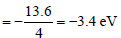Energy of second excited state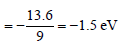Difference between ground state and 2nd
excited state = 13.6 – 1.5 = 12.1 eV
So, electron can be excited upto 3rd orbit
No. of possible transition
1 → 2, 1 → 3, 2 → 3
So, three lines are possible

QUESTION: 27

The total energy of electron in the ground stateof hydrogen atom is – 13.6 eV. The kinetic energyof an electron in the first excited state is        

Solution:

Energy in the first excited state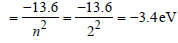But K.E. = –(Total energy) = +3.4 eV.

QUESTION: 28

The ground state energy of hydrogen atom is 13.6eV. When its electron is in the first excited state, its excitation energy is 

Solution:

When the electron is in first excited state
(n = 2), the excitation energy is given by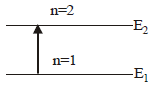ΔE = E2 – E1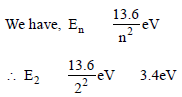Given E1 = –13.6eV
∴Δ E = ( – 3.4) – ( – 13.6) = 10.2 eV.

QUESTION: 29

In a Rutherford scattering experiment when a projectile of charge Z1 and mass M1 approaches a target nucleus of charge Z2 and mass M2, the distance of closest approach is r0. The energy of the projectile is            

Solution:

The kinetic energy of the projectile is given by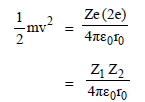Thus energy of the projectile is directly
proportional to Z1, Z2

QUESTION: 30

The ionization energy of the electron in thehydrogen atom in its ground state is 13.6 eV.The atoms are excited to higher energy levels toemit radiations of 6 wavelengths. Maximumwavelength of emitted radiation corresponds tothe transition between 

Solution: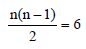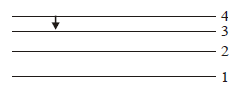n2 – n – 12 = 0
(n – 4) (n + 3) = 0 or n = 4

QUESTION: 31

The energy of a hydrogen atom in the groundstate is – 13.6 eV. The energy of a He+ ion in thefirst excited state will be 

Solution:

Energy of a H-like atom in it's nth state is
given by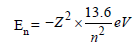For, first excited state of He+, n = 2, Z = 2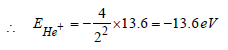QUESTION: 32

An alpha nucleus of energy 1/2mv2  bombards a heavy nuclear target of charge Ze. Then the distance of closest approach for the alpha nucleus will be proportional to 

Solution:

Kinetic energy of alpha nucleus is equall to
electrostatic potential energy of the system
of the alpha particle and the heavy nucleus.
That is,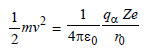where r0 is the distance of closest approach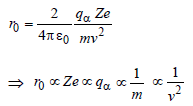Hence, correct option is (c).

QUESTION: 33

The wavelength of the first line of Lyman seriesfor hydrogen atom is equal to that of the secondline of Balmer series for a hydrogen like ion. Theatomic number Z of hydrogen like ion is 

Solution:

For first line of Lyman series of hydrogen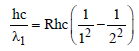For second line of Balmer series of hydrogen
like ion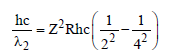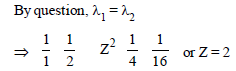QUESTION: 34

An electron in the hydrogen atom jumps from excited state n to the ground state. The wavelength so emitted illuminates a
photosensitive material having work function 2.75 eV. If the stopping potential of thephotoelectron is 10 V, the value of n is [2011 M]

Solution: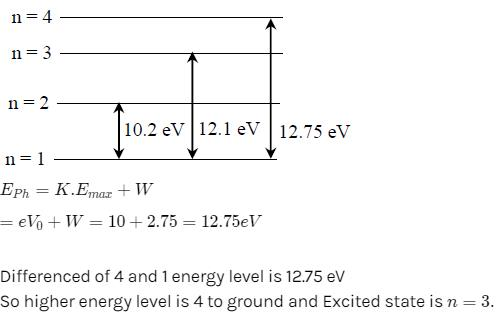QUESTION: 35

Out of the following which one is not a possibleenergy for a photon to be emitted by hydrogenatom according to Bohr’s atomic model? [2011M]

Solution:

Obviously, difference of 11.1eV is not
possible.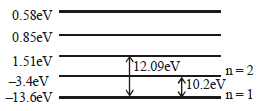QUESTION: 36

Electron in hydrogen atom first jumps from third excited state to second excited state and then from second excited to the first excited state.The ratio of the wavelength λ1 : λ2 emitted inthe two cases is 

Solution: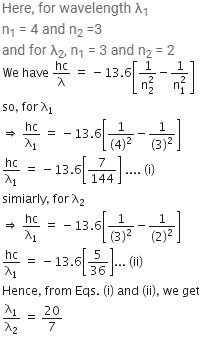QUESTION: 37

An electron of a stationary hydrogen atom passes from the fifth energy level to the ground level. The velocity that the atom acquired as a result of photon emission will be : 

(m is the mass of the electron, R, Rydberg constant and h Planck’s constant)

Solution:

For emission, the wave number of the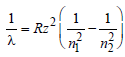R= Rydberg constant, Z = atomic number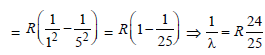linear momentum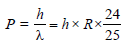(de-Broglie hypothesis)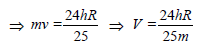QUESTION: 38

The transition from the state n = 3 to n = 1 in ahydrogen like atom results in ultravioletradiation. Infrared radiation will be obtained inthe transition from : [2012M]

Solution:

The frequency of the transition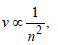when n = 1, 2, 3.

QUESTION: 39

The ratio of longest wavelengths corresponding to Lyman and Blamer series in hydrogen spectrum is [NEET 2013]

Solution:

For Lyman series (2 → 1)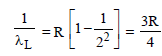For Balmer series (3 → 2)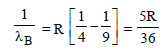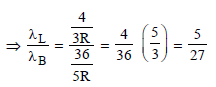QUESTION: 40

An electron in hydrogen atom makes a transition n1 → n2 where n1 and n2 are principal quantum numbers of the two states. Assuming Bohr’s model to be valid the time period of the electron in the initial state is eight times that in the final state. The possible values of n1 and n2 are        [NEET Kar. 2013]

Solution:

T ∝ n3
Tn1 = 8 Tn2 (given)
Hence, n1 = 2n2